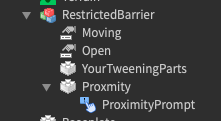# ProximityPrompt Group Rank Barrier

So I’ve been trying to create a boom barrier that is only restricted to certain group ranks however I am unsure how to approach this, I have gotten the backbone of the script which functions the barrier however not the actual part where it states group ranks.
This is what I have got so far.

``````local model = script.Parent
local Centre = script.Parent.PrimaryPart
function move()
if model.Open.Value == false and model.Moving.Value == false then
model.Open.Value = true
model.Moving.Value = true
for i = 1,70 do
wait(0.01)
end
model.Moving.Value = false
elseif model.Open.Value == true and model.Moving.Value == false then
model.Moving.Value = true
for i = 1,70 do
wait(0.01)
end
model.Open.Value = false
model.Moving.Value = false
else

end
end

function trigger(m)
model.Trigger.Value = true
move()
model.Trigger.Value = false
end

script.Parent.Parent.ButtonPart.ProximityPrompt.Triggered:connect(trigger)
``````

Here is a video of the current script in action

Any help would be greatly appreciated.

3 Likes

You can try using GetRankInGroup or GetRoleInGroup to determine which rank/role is allowed to interact with the barrier.

How would I got about saying to cancel the trigger of the barrier though? Cause the barrier would still go up?

How did you implement the group restrictions? It should work depending on their ranks.

For example:

``````local groupid = ... --Your group id

if Player:GetRankInGroup(groupid) == x then --where x is your rank number
--Play the tween here
else
print("Cannot open barrier")
end
``````

But if that does not work. Then one solution I can think of is that you can set up extra measures to determine whether the player has a certain Value checked.

For example:

``````local groupid = ... --Your group id

local isACertainRank = instance.new("BoolValue")
isACertainRank.Parent = Player
isACertainRank.Name = "RankName"

if Player:GetRankInGroup(groupid) == x then --Where x is your rank number
isACertainRank.Value = true
end
end)
``````

Then have your prompt detect whether the player has this bool checked or not. If it’s checked, play the tween, if not, do nothing.

If you’re talking about cancelling the tween mid-way after it’s been interacted. Then maybe you can store the CFrame values of the barrier (both initial and final) in a table and referencing it from there. I don’t work too much on CFrame as I mostly use TweenService to do my tweening.

I’ve tried something along the lines of this, however it still doesn’t seem to work.

``````game.Players.PlayerAdded:Connect(function(Player)
if Player:GetRankInGroup(10051621) == 255 then
model.Open.Value == true and model.Moving.Value == true
else model.Open.Value == false and model.Moving.Value == false
end
``````

I’ve also tried something like this, at this point I am very confuzzled.

``````game.Players.PlayerAdded:Connect(function(Player)

end)
if game.players:GetRankInGroup(10051621) == 255 then
script.Parent.Parent.ButtonPart.ProximityPrompt.Triggered:connect(trigger)
else
print("Could not open gate")
end
``````

I assume you mean the barrier will not tween, the GetRankInGroup is not registering the player, or both.

In that case, did you set up your proximity prompt correctly? I’d imagine that it’s the proximity prompt that’s the issue.

Here’s an example explaining your issue:``````local PPS = game:GetService("ProximityPromptService")

PPS.PromptTriggered:Connect(function(Prompt,Player)
-- In case your want to use the player's character/humanoid
local Character = Player.Character
local Humanoid = Character:WaitForChild("Humanoid")

-- Because a specific prompt is triggered
-- path to your model, and detect whether it's the correct barrier
-- you can wrap this into a pcall function to avoid breaking your script
if Prompt.Parent.Parent.Name == "RestrictedBarrier" then

-- After it detects the correct barrier, reference the model and its values
local Barrier = Prompt.Parent.Parent
local Moving = Barrier.Moving
local Open = Barrier.Open

-- From here, detect whether the player is in a certain rank to open the barrier
if Player:GetRankInGroup(10051621) == 255 then
if not Moving.Value and not Open.Value then
Moving.Value = true
Open.Value = true
-- Tween your parts to open here
-- and after your tween, change the Moving.Value back to false
Moving.Value = false
elseif not Moving.Value and Open.Value then
Moving.Value = true
Open.Value = false
-- Tween your parts to close here
-- and after your tween, change the Moving.Value back to false
Moving.Value = false
end
else
print("Lacking permission")
-- If the player does not have the permission to open the barrier
-- then do whatever you want to them here
-- (e.g.: Teleport them a certian distance away from the barrier)
end
end
end)
``````

This has solved the issue, cheers for the help.

1 Like